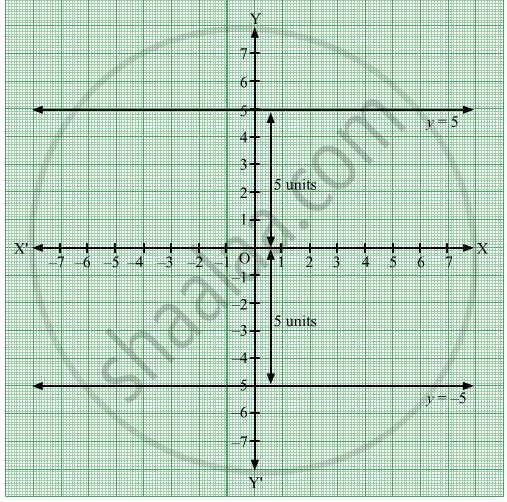Share

# How Many Lines Are There Which Are Parallel to X-axis and Having a Distance 5 Units? - Geometry

ConceptEquations of Lines Parallel to the X-axis and Y-axis

#### Question

How many lines are there which are parallel to X-axis and having a distance 5 units?

#### Solution

The equations of the lines parallel to X-axis and at a distance 5 units from it are y = 5 and y = −5.Thus, there are two lines which are parallel to X-axis and having a distance 5 units from it.

Is there an error in this question or solution?

#### APPEARS IN

Balbharati Solution for Balbharati Class 9 Mathematics 2 Geometry (2019 to Current)
Chapter 7: Co-ordinate Geometry
Problem Set 7 | Q: 6 | Page no. 99
Solution How Many Lines Are There Which Are Parallel to X-axis and Having a Distance 5 Units? Concept: Equations of Lines Parallel to the X-axis and Y-axis.
S